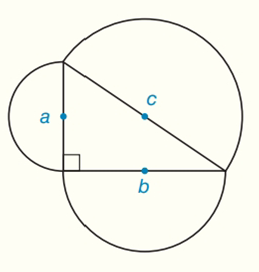Chapter 8.CR, Problem 38CRElementary Geometry For College St...

7th Edition
Alexander + 2 others
ISBN: 9781337614085

Solutions

Chapter
SectionElementary Geometry For College St...

7th Edition
Alexander + 2 others
ISBN: 9781337614085
Textbook Problem

Prove that if semicircles are constructed on each of the sides of a right triangle, then the area of the semicircle on the hypotenuse is equal to the sum of the areas of the semicircles on the two legs.To determine

To prove:

If semicircles are constructed on each of the sides of a right triangle, then the area of the semicircle on the hypotenuse is equal to the sum of the areas of the semicircles on the two legs.

Explanation

Formula:

The area of the semi-circle A=12πr2

Pythagorean Theorem:

Regardless of what figure we construct on the sides of the right triangle, as long as the figures are similar, the sum of the areas constructed on the shorter sides (the legs) would equal the area of the square on the longer side (the hypotenuse) then we will always get back to a2+b2=c2.

Calculation:

Consider the following diagram:

To find the area of the semi-circle with diameter b:

Area of the semi-circle A=12πr2

A=12π(b2)2=12π×b24=πb28

The area of the semi-circle with diameter b = πb28

To find the area of the semi-circle with diameter a:

A=12π(a2)2=12π×a24=πa28

To find the area of the semi-circle with diameter c:

Still sussing out bartleby?

Check out a sample textbook solution.

See a sample solution

The Solution to Your Study Problems

Bartleby provides explanations to thousands of textbook problems written by our experts, many with advanced degrees!

Get Started

Find f. f(x) = ex 2 sin x, f(0) = 3, f(/2) = 0

Single Variable Calculus: Early Transcendentals, Volume I

In Problems 1-16, use integration by parts to evaluate the integral. 11.

Mathematical Applications for the Management, Life, and Social Sciences

Sometimes, Always, or Never: If an ≥ bn ≥ 0 for all n and {bn} diverges, then {an} diverges.

Study Guide for Stewart's Single Variable Calculus: Early Transcendentals, 8th